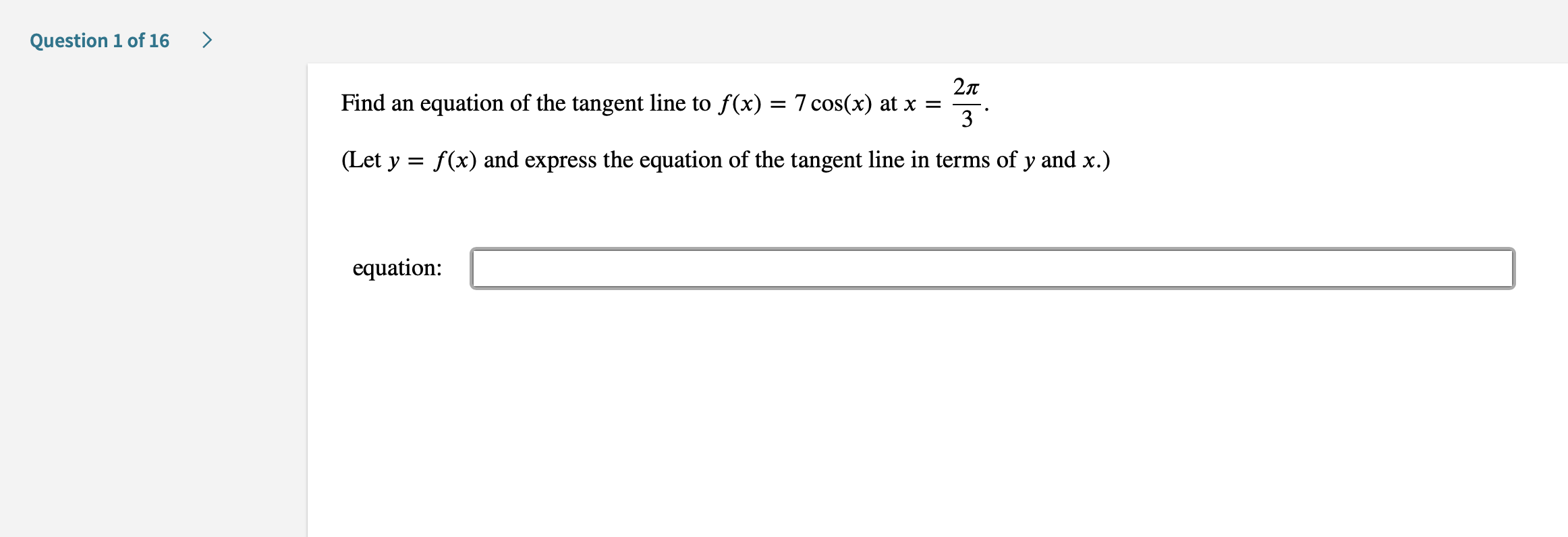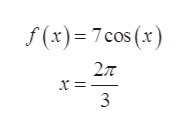>Question 1 of 1627TFind anequation of the tangent line to f(x) = 7 cos(x) at x =3(Let y f(x) and express the equation of the tangent line in terms of y and x.)equation

Questionhelp_outlineImage Transcriptionclose> Question 1 of 16 27T Find an equation of the tangent line to f(x) = 7 cos(x) at x = 3 (Let y f(x) and express the equation of the tangent line in terms of y and x.) equation fullscreen
Step 1

To determine the equation of the tangent line.

Step 2

Given:help_outlineImage Transcriptionclosef(x)7 cos (x) = 7 x = _ 3 fullscreen
Step 3

The equation of tangent line to a function at a poi...

Want to see the full answer?

See Solution

Want to see this answer and more?

Our solutions are written by experts, many with advanced degrees, and available 24/7

See Solution
Tagged in

Derivative